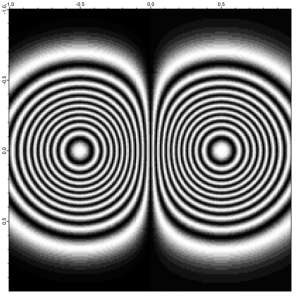# Display images in interferogram (phase variation) mode

The ModifyImage operation includes a lookup wave option allowing the use of a non-linear color mapping. It occurred to me that this might be a very simple way to display surface heights or phase changes in a manner similar to that created by optical interferometers. All one has to do is make the appropriate sinusoidal lookup wave, and match it to the desired scale. In the example provided, the amplitude of the image wave is measured in wavelengths. For other units, you will have to alter the scaling. The displayed image somehat resembles a conventional contour plot, but has some phase/sign ambiguity. It used an input amplitude of 5.25 .

#pragma rtGlobals=1     // Use modern global access method.
//  best to try this in a new Igor experiment

function MakeAndShowTestImage(a)
variable//  wave peak amplitude in this example, in wavelengths

make/O/N=(200,200) w2D
setscale x, -1, 1,"" w2D
setscale y, -1, 1,"" w2D
// insert (replace) your definition of w2D here:
// height of w2D is measured in wavelengths
w2D  = a*(exp(-(((x-0.5)/0.4)^2 + (y/0.4)^2)) - exp(-(((x+0.5)/0.4)^2 + (y/0.4)^2)))

DoWindow Graph0
if(!V_flag) //  if Graph0 doesn't exist, create it with new image
newimage w2D
ModifyGraph height={Plan,1,left,top}
endif
MakeNonLinearColorFunc(w2D) //  make the lookup wave
ModifyImage/W=Graph0 w2D ctab= {*,*,Grays256,0},  lookup = wNL
end
//-------------------------------------------------------------------------------------------------------
function MakeNonLinearColorFunc(inwave)
// make a nonlinear image LOOKUP wave to simulate interference fringes
wave inwave //  the 2D image wave to be shown as an interferogram

make/O/N=500 wNL
variable xmin = waveMin(inwave), xmax = waveMax(inwave)
setscale/I x, xmin, xmax,"" wNL
wNL = (cos(2*pi*(xmax-x)))^2    //  set brightest color at maximum of image
endForumSupportGallery